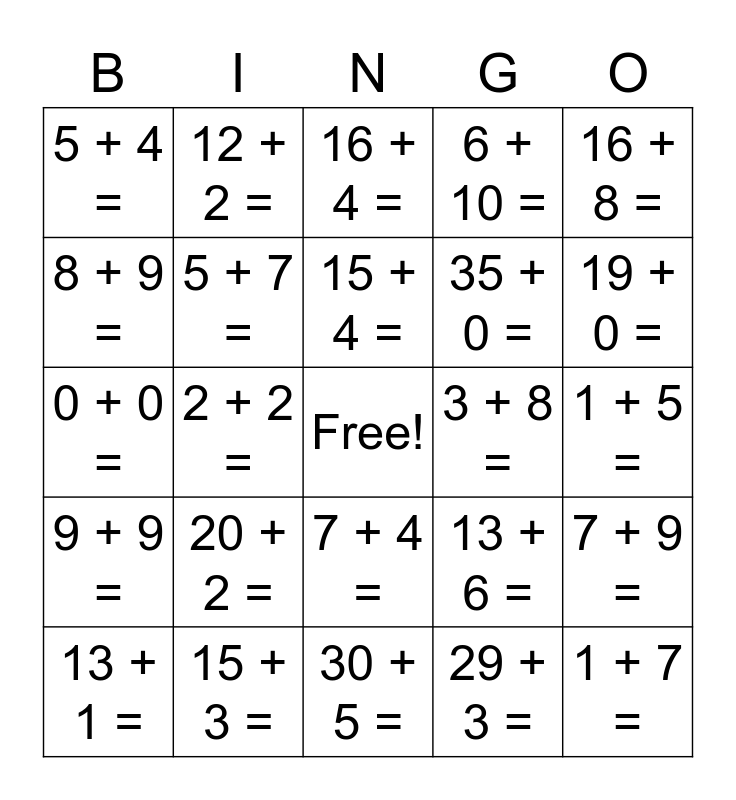# Math!This bingo card has a free space and 50 words: 10 + 1, 1 + 7 =, 4 + 3 =, 30 + 5 =, 20 + 2 =, 6 + 6 =, 12 + 2 =, 2 + 8 =, 8 + 9 =, 20 + 4 =, 5 + 7 =, 6 + 10 =, 7 + 9 =, 13 + 6 =, 8 + 4 =, 33 + 4 =, 15 + 4 =, 3 + 8 =, 12 + 7 =, 16 + 8 =, 2 + 2 =, 29 + 3 =, 5 + 4 =, 35 + 0 =, 28 + 2 =, 1 + 1 =, 19 + 0 =, 31 + 4 =, 16 + 4 =, 7 + 4 =, 6 + 3 =, 2 + 2 =, 34 + 2 =, 8 + 5 =, 15 + 3 =, 14 + 2 =, 30 + 4 =, 12 + 4 =, 5 + 6 =, 1 + 5 =, 38 + 1 =, 26 + 2 =, 13 + 1 =, 11 + 0 =, 13 + 1 =, 0 + 0 =, 23 + 6 =, 13 + 6 =, 9 + 9 = and 2 + 3 =.

⚠ This card has duplicate items: 13 + 6 = (2), 2 + 2 = (2), 13 + 1 = (2)

## Play Online